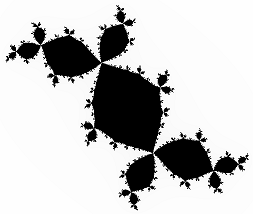# Visual Basic 2010 (Windows) GuideFractals: Julia SetsJulia Set images

When we plot the Mandelbrot set, we use a starting value of z equal to zero. The value of the complex number c varies each time we call the IsInMandelbrotSet() procedure.

For a Julia Set, the value of c remains constant regardless of the starting pixel position. Instead, the value of z is taken from the pixel position being plotted.

In order to plot a Julia Set, you need to allow the user to be able to specify the real and imaginary parts of the complex number c.

The following algorithm should work for you.

```Public Function IsInJuliaSet(a As Double, b As Double, zx As Double, zy As Double, maxit As Integer) As Integer    Dim squareOfDistance As Double    Dim xSquare As Double = 0.0    Dim ySquare As Double = 0.0    Dim startA As Double = a    Dim startB As Double = b    Dim numIterations As Integer = 0    While numIterations < maxit       xSquare = zx * zx       ySquare = zy * zy       squareOfDistance = xSquare + ySquare          If squareOfDistance > 4 Then          Return numIterations       End If       zy = 2 * zx * zy + startB       zx = (xSquare - ySquare) + startA       numIterations += 1    End While    Return numIterations End Function```

Obviously you need to tweak the application to accomodate this code. When you have, the next thing you need are some values for c that produce interesting images. Try the following,

```c = -0.12375 + 0.56508i c = -0.12 + .74 i c = -0.11 + 0.6557i c = -0.194 + 0.6557i c = -0.125 + 0i```

Other images can be produced with experimentation or by looking on the WWW.

The Julia Sets are related to the Mandelbrot set. One thing you may wish to add to your application is the ability to use the value of c from any point on the image to draw the corresponding Julia Set. In our application, a right mouse click might suffice.